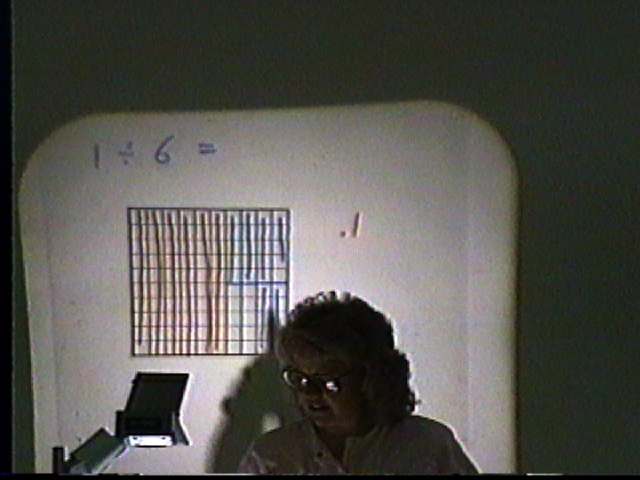Home
 Division .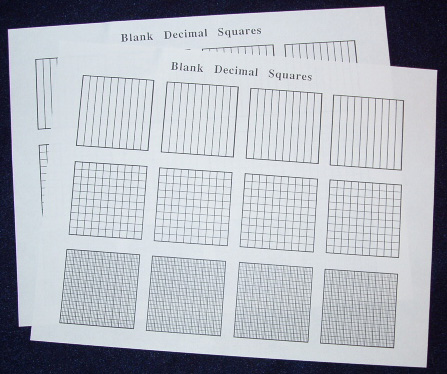Materials: A sheet of Blank Decimal Squares with Tenths Hundredths and Thousandths for each student . There are two concepts of division the Measurement Concept and the Sharing Concept and both are used in the following illustrations to make sense of the given examples involving decimals. . Measurement Concept Place red squares with 2 shaded parts and 8 shaded parts on the overhead and ask how many times the smaller shaded amount fits into the larger shaded amount. Repeat this activity for: green squares with 75 and 15 shaded parts; and yellow squares with 675 and 25 shaded parts. Point out that this use of division comparing two amounts is called the "measurement concept".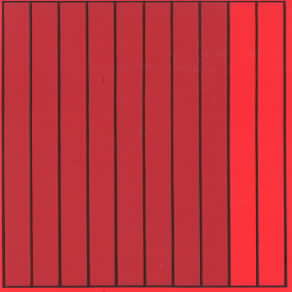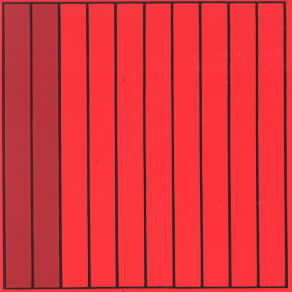.8 ÷ .2 = 4 . Activity - Shade Blank Decimal Squares to illustrate the following operations: .24 ÷ .04; ..675 ÷ .025; and 1.5 ÷ .3. Then use an algorithm for dividing one decimal by another. . Discuss the methods used to obtain the answers. Blank Decimal Squares can be used to show how many times one amount fits into the other by shading and measuring off (marking off) squares. This visual approach shows that we need to determine how many times 25 parts fit into 675 parts. Write the long division algorithm for dividing the whole numbers 675 by 25 on the overhead and then write the algorithm for dividing the decimals .675 by .025.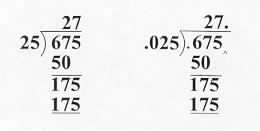Note that these these algorithms are very similar. In fact when dividing one decimal by another where both have the same number of decimal places we first move decimal points so that we are actually dividing one whole number by another. Also discuss the fact that one of the major concepts of division is determining how many times larger one amount is than another whether the amounts are represented by whole numbers fractions or decimals. Sharing Concept Place a green transparent square with 45 shaded parts on the overhead and ask how much each person would receive of the shaded part if it was divided evenly among 3 people. Point out that this use of division is called the "sharing concept"..45 ÷ 3 = .15 Activity - Shade Blank Decimal Squares to illustrate the following operations: .28 ÷ 4; .350 ÷ 5; and .70 ÷ 10. Then obtain the quotients by using an algorithm for division. discuss the results. These students below are selecting a square rolling a die and using the sharing concept of division to compute quotients. The student on the left selected a green square with 40 shaded parts out of 100 and rolled a 4 on the die. Since 40 ÷ 4 = 10 she knows that .40 ÷ 4 = .10. The other student selected a green square with 70 shaded parts out of 100 and rolled a 6 on her die. since 70 ÷ 6 is 12 to the nearest whole number of parts on a green square ÷ 6 = .12 to the nearest hundredth.Photo courtesy of Herb Moyer The sharing concept can also be used to illustrate division of one whole number by another. In the following demonstration the student is attempting to divide a square into 6 equal parts with 4 parts to show that 1 ÷ 6 is approximately .16. At this point in the demonstration she has obtained 6 groups of 14 hundredths each and has 10 hundredths left to continue the division process.